# n元函数局部极值存在的一阶充分条件*

(重庆理工大学 理学院， 重庆 400054)

## 引 言

min(max) f(x), xRn,

(1)

f(x)≥(>)f(x0), ∀xU, xx0,

f是一元函数时,问题(1)的极值点是否存在的一阶充分条件如下:

(a) 如果当x∈(x0-δ,x0)时, f′(x)<0,而当x∈(x0,x0+δ)时, f′(x)>0,则f(x)在x0取得极小值;

(b) 如果当x∈(x0-δ,x0)时, f′(x)>0,而当x∈(x0,x0+δ)时, f′(x)<0,则f(x)在x0取得极大值;

(c) 如果当x∈N0(x0,δ)时f′(x)的符号不变,则fx0不取得极值,其中f′(x)表示fx0的导数

f是多元函数时,问题(1)的极值是否存在的二阶充分条件如下:

n=2时,该定理退化为二元函数极值是否存在的二阶充分条件

(a) AC-B2>0时有极值,且当A<0时有极大值,当A>0时有极小值;

(b) AC-B2<0时无极值;

(c) AC-B2=0时可能有极值,可能无极值,还需另做讨论

## 1 预 备 知 识

f[λx+(1-λ)y]≤(≥)max(min){f(x), f(y)},

## 2 一阶充分条件

min(max) f(x), xS,

(2)

(a) 若存在x0的一个邻域U使得

(f(x))T(x-x0)≥0, ∀xUS\{x0}，

fx0取得极小值;

(b) 若存在x0的一个邻域U使得

(f(x))T(x-x0)≤0, ∀xUS\{x0},

fx0取得极大值;

(c) 若存在两个不同的方向uRn, vRn, u0,v0,使得对任意充分小的t>0,(f(x0+tu))Tu>0(<0)且(f(x0+tv))Tv<0(>0),则fx0不取得极值

(a) 对假设的U,任取点xUS\{x0}由引理2,存在点y=λx+(1-λ)x0,某λ∈(0,1),使得

f(x)-f(x0)=(f(y))T(x-x0)

(3)

U是开凸集,由引理1,US是开凸集所以yUS

(f(y))T(y-x0)≥0,

λ(f(y))T(x-x0)≥0,

(f(y))T(x-x0)≥0

(b) 类似可证

(c) 不妨假定对任意充分小的t>0,(f(x0+tu))Tu>0,(f(x0+tv))Tv<0任取某一充分小的t>0,由引理2,存在0<t′<t使得

f(x0+tu)=f(x0)+t(f(x0+tu))Tu

f(x0+tu)>f(x0)

(4)

f(x0+tv)<f(x0)

(5)

f(x0+tv)<f(x0)<f(x0+tu)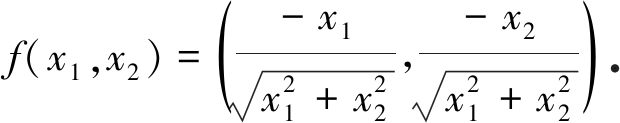(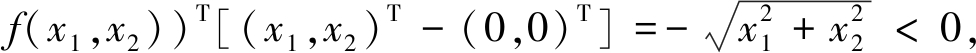∀(x1,x2)∈US\(0,0)

(f(x1,x2))T[(x1,x2)T-(0,0)T]=(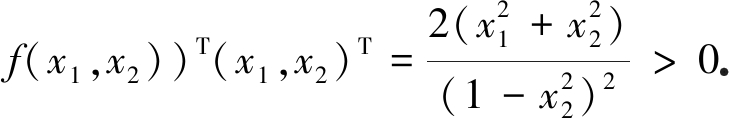(f(x0+tu))Tu=6t5>0, (f(x0+tv))Tv=-6t5<0

(f(x1,x2))T[(x1,x2)T-(0,0)T]=0,

∀(x1,x2)∈US,(x1,x2)≠(0,0)

(f(x1,x2,x3))T[(x1,x2,x3)T-(0,0,0)T]=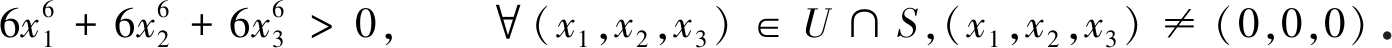(a) 若存在x0的某一邻域U,使得f′(x)(x-x0)>0,∀xSU,xx0,也就是当x<x0 时, f′(x)<0,当x>x0 时, f′(x)>0,所以得定理1(a);

(b) 同理这是定理1中的(b);

(c) 由于xUS, x<x0xUS, x>x0时, f′(x)的符号相同, 所以得定理1(c)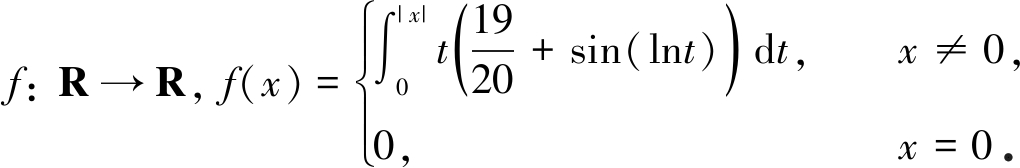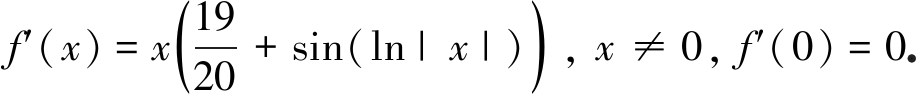f′(pk)(pk-0)=f′(pk)pk>0

f′(qk)(qk-0)=f′(qk)qk<0

(a) 设fS上拟凸，则fx0取得极小值当且仅当存在x0的一个邻域U使得

(f(x))T(x-x0)≥0, ∀xUS\{x0};

(b) 设fS上拟凹，则fx0取得极大值当且仅当存在x0的一个邻域U使得

(f(x))T(x-x0)≤0, ∀xUS\{x0}

(a) 设x0fS上的极小点,则存在x0 的某一邻域U,使得

f(x)≥f(x0), ∀xUS, xx0

(b) 同理可证

## 3 结 论

 华东师范大学数学系. 数学分析(下册)[M]. 第4版. 北京: 高等教育出版社, 2010.(Department of Mathematics, East China Normal University. Mathematical Analysis(Vol 2)[M]. 4th ed. Beijing: Higher Education Press, 2010.(in Chinese))

 BAZARRA M S. 非线性规划: 理论与算法[M]. 张春柏, 王化存, 译. 贵阳: 贵州人民出版社, 1986.(BAZARRA M S. Nonlinear Programming Theory and Algorithm[M]. ZHANG Chunbai, WANG Huacun, transl. Guiyang: Guizhou People’s Publishing Press, 1986.(in Chinese))

 HIRIART-URRUTY J B, STRODIOT J J, NGUEN H V. Generalized Hessian matrix and second order optimality conditions for problems with C1,1 data[J]. Applied Mathematics and Optimization, 1984, 11: 43-56.

 BEDNARIK D, PASTOR K. l-stable functions are continuous[J]. Nonlinear Analysis: Theory, Methods & Applications, 2009, 70: 2317-2324.

 BEN-TAL A, ZOW J. Directional derivatives in nonsmooth optimization[J]. Journal of Optimization Theory and Applications, 1985, 47: 483-490.

 GINCHEV I, GUERRAGGIO A, ROCCA M. From scalar to vector optimization[J]. Applications of Mathematics, 2006, 51: 5-36.

 PAN L, XIU N, ZHOU S. On solutions of sparsity constrained optimization[J]. Journal of the Operations Research Society of China, 2015, 3: 421-439.

 YAMAMOTO S, KUROIWA D. Constraint qualifications for KKT optimality condition in convex optimization with locally Lipschitz inequalty constraints[J]. Nonlinear Analysis, 2016, 2: 101-116.

 MOVAHEDIAN N, NOBAKHTIAN S, SARABADAN M. Nonsmooth sparsity constrained optimization problems: optimality conditions[J]. Optimization Letters, 2019, 13(5): 1027-1038.

 MOVAHEDIAN N. Scaled constraint qualifications for generalized equation constrained problems and application to nonsmooth mathematical programs with equilibrium constraints[J]. Positivity, 2019, 24: 253-285.

 HUANG L R, NG K F. On some relations between Chaney’s generalized second-order directional derivative and that of Ben-Tal and Zow[J]. SIAM Journal on Control and Optimization, 1996, 34(4): 1220-1234.

 BEDNARIK D, PASTOR K. Elimination of strict convergence in optimization[J]. SIAM Journal on Control and Optimization, 2004, 4(3): 1063-1077.

 GINCHEV I. Higher order optimality conditions in nonsmooth optimization[J]. Optimization, 2002, 51(1): 47-72.

 HUANG L R, NG K F. Second-order necessary and sufficient conditions in nonsmooth optimization[J]. Mathematical Programming, 1994, 66: 379-402.

 HUANG L R. Separate necessary and sufficient conditions for the local minimum of a function[J]. Journal of Optimization Theory and Applications, 2005, 125: 241-246.

 COMINETTI R, CORREA R. A generalized second-order derivative in nonsmooth optimization[J]. SIAM Journal on Control and Optimization, 1990, 28: 789-809.

 CHAN W L, HUANG L R, NG K F. On generalized directional derivatives and Taylor expansions in nonsmooth optimization[J]. SIAM Journal on Control and Optimization, 1994, 32(3): 591-611.

 ROCKFELLA R T. First- and second-order epi-differentiability in nonlinear programming[J]. Transactions of the American Mathematical Society, 1998, 307: 75-108.

 JIMENEZ B, NOVO V. Higher-order optimality conditions for strict local minima[J]. Annals of Operations Research, 2008, 157: 183-192.

 BEDNARIK D, PASTOR K. On second-order conditions in unconstrained optimization[J]. Mathematical Programming, 2008, 113: 283-289.

 BAIER R, FARKHI E, ROSHCHINA V. Directed subdifferentiable functions and the directed subdifferential without delta-convex structure[J]. Journal of Optimization Theory and Applications, 2014, 160(2): 391-414.

 BAIER R, FARKHI E, ROSHCHINA V. From quasidifferentiable to directed subdifferentiable functions: exact calculus rules[J]. Journal of Optimization Theory and Applications Volume, 2016, 171: 384-401.

 SISARAT N, WANGKEEREE R. Characterizing the solution set of convex optimization problems without convexity of constraints[J]. Optimization Letters, 2019, 2: 1-18.

 HUBBARD J H, HUBBARD B B. Vector Calculus, Linear Algebra, and Differential Forms: a Unified Approach[M]. 3rd ed. New York: Matrix Editions, 2013.

# First-Order Sufficient Conditions for Existence of Local Extremums of Multivariate Functions

HUANG Zhenggang

(College of Science, Chongqing University of Technology, Chongqing 400054, P.R.China)

Abstract: The unified 1st-order sufficient condition was proposed for existence of the local extremums of n-variable functions, in a case more general than classical unconstrained optimization ones. The difficulty of no such 1st-order sufficient condition in optimization theories was solved. Moreover, the 1st-order sufficient condition for 1-variable functions was proved to be a special case of the results. The work can eliminate the shortages of the 2nd-order sufficient conditions for existence of local extremums of classical multivariate functions, and the result is both necessary and sufficient under the assumption of quasiconvexity or quasiconcavity.

Key words: local extremum; 1st-order sufficient condition; 2nd-order sufficient condition; quasiconvexity; quasiconcavity

*收稿日期： 2019-08-09； 修订日期：2020-04-21

DOI: 10.21656/1000-0887.400237

Foundation item: The National Natural Science Foundation of China(50573095)